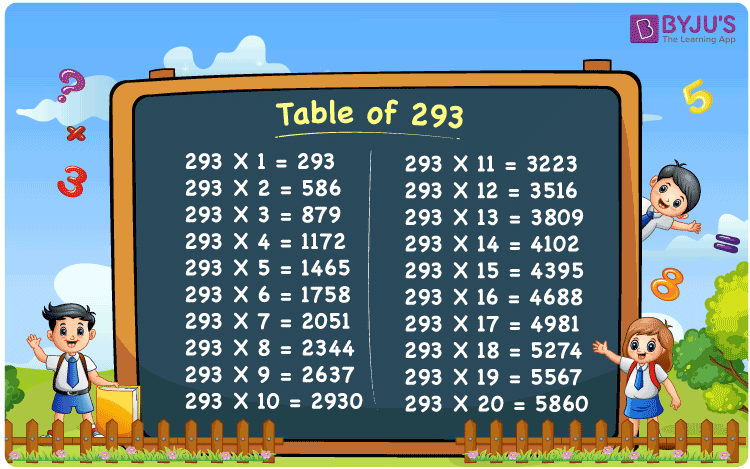Checkout JEE MAINS 2022 Question Paper Analysis : Checkout JEE MAINS 2022 Question Paper Analysis :

# Table of 293

The table of 293 is the multiplication table which represents the values obtained when the number 293 is multiplied by a set of consecutive natural numbers. These values also define the multiples of 293. Repeated addition of number 293 to itself also represents 293 times table. Learn more multiplication tables at BYJU’S. Also, students can access the table of 293 in PDF format for free here.## What is the 293 Times Table?

The table of 293 is not an easy table to memorise. Hence, students must learn the tricks to create multiplication tables. Follow the chart below, which shows the multiplication table of 293 in both multiplication and addition form.

 293×1 = 293 293 293×2 = 586 293 + 293 = 586 293×3 = 879 293 + 293 + 293 = 879 293×4 = 1172 293 + 293 + 293 + 293 = 1172 293×5 = 1465 293 + 293 + 293 + 293 + 293 = 1465 293×6 = 1758 293 + 293 + 293 + 293 + 293 + 293 = 1758 293×7 = 2051 293 + 293 + 293 + 293 + 293 + 293 + 293 = 2051 293×8 = 2344 293 + 293 + 293 + 293 + 293 + 293 + 293 + 293 = 2344 293×9 = 2637 293 + 293 + 293 + 293 + 293 + 293 + 293 + 293 + 293 = 2637 293×10 = 2930 293 + 293 + 293 + 293 + 293 + 293 + 293 + 293 + 293 + 293 = 2930

## Multiplication Table of 293

Learn the table of 293 up to 20 times from the chart below.

 293 × 1 = 293 293 × 2 = 586 293 × 3 = 879 293 × 4 = 1172 293 × 5 = 1465 293 × 6 = 1758 293 × 7 = 2051 293 × 8 = 2344 293 × 9 = 2637 293 × 10 = 2930 293 × 11 = 3223 293 × 12 = 3516 293 × 13 = 3809 293 × 14 = 4102 293 × 15 = 4395 293 × 16 = 4688 293 × 17 = 4981 293 × 18 = 5274 293 × 19 = 5567 293 × 20 = 5860

## Solved Example on the Table of 293

Example 1:

Amrit earns Rs 293 in a day. Calculate how much he would earn in 20 days.

Solution:

Money earned by Amrit in a day = Rs 293

Amount earned by him in 20 days = 293 x 20 = 5860

Therefore, Amrit earns Rs 5860 in 20 days.

## Frequently Asked Questions on Table of 293

### What is 293 multiplied by 17 equal to?

293 multiplied by 17 is equal to 4981.

### What is the sum of 8th and 15th multiple of 293?

From the table of 293,
293 x 8 = 2344.
293 x 15 = 4395.
2344 + 4395 = 6739.

### Estimate 293 times 11 plus 19 minus 6.

293 times 11 = 293 x 11 = 3223.
293 times 11 plus 19 minus 6 = 3223 + 19 – 6 = 3236.